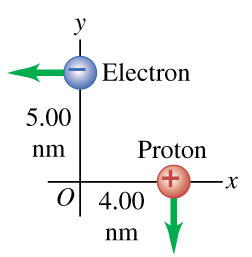# Problem: An electron and a proton are each moving at 795 km/s in perpendicular paths as shown in (Figure 1) .Part AAt the instant when they are at the positions shown, find the magnitude of the total magnetic field they produce at the origin.Part BFind the direction of the total magnetic field they produce at the origin.

###### FREE Expert Solution

Magnetic field:

$\overline{){\mathbf{B}}{\mathbf{=}}\frac{{\mathbf{\mu }}_{\mathbf{0}}\mathbf{qvs}\mathbf{i}\mathbf{n}\mathbf{\theta }}{\mathbf{4}\mathbf{\pi }{\mathbf{r}}^{\mathbf{2}}}}$

Part A

The total magnetic field at the origin:

85% (169 ratings)###### Problem Details

An electron and a proton are each moving at 795 km/s in perpendicular paths as shown in (Figure 1) .Part A

At the instant when they are at the positions shown, find the magnitude of the total magnetic field they produce at the origin.

Part B

Find the direction of the total magnetic field they produce at the origin.